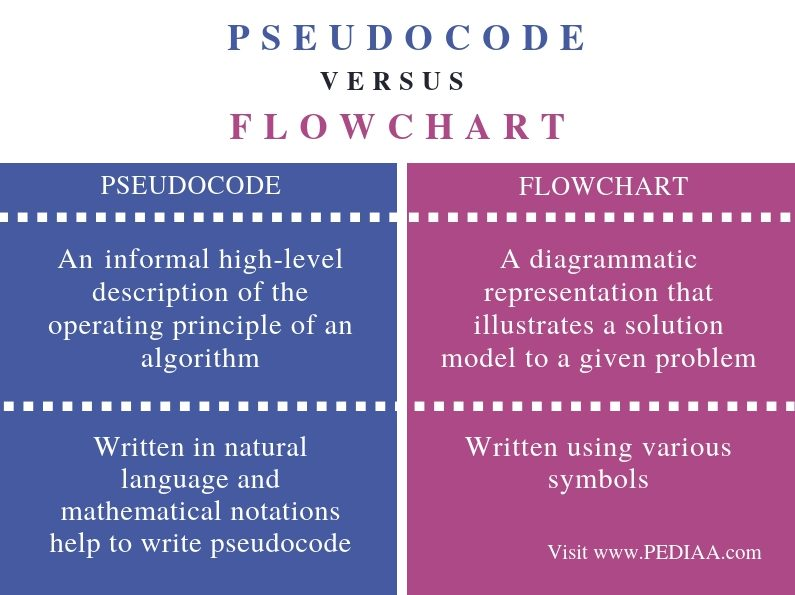# What is the Difference Between Pseudocode and Flowchart

The main difference between Pseudocode and Flowchart is that pseudocode is an informal high-level description of an algorithm while flowchart is a pictorial representation of an algorithm.

An algorithm is a step by step sequence of solving a given problem. There can be several approaches to solve a problem. Further, a computer program is a set of instructions given to the computer to perform a certain task. Therefore, algorithms are used in computer programming. Here, time complexity refers to the time required to run an algorithm while space complexity is the amount of memory necessary for an algorithm. Moreover, it is important to select the best algorithm to solve it after analyzing the time complexity and space complexity. Thus, pseudocode and flowchart are two methods of representing an algorithm.

### Key Areas Covered

1. What is a Pseudocode
– Definition, Functionality
2. What is a Flowchart
– Definition, Functionality
3. What is the Difference Between Pseudocode and Flowchart
– Comparison of Key Differences

### Key Terms

Algorithm, Flowchart, Pseudocode## What is a Pseudocode

A pseudocode is an informal way of writing a program.  However, it is not a computer program. It only represents the algorithm of the program in natural language and mathematical notations. Besides, there is no particular programming language to write a pseudocode. Unlike in regular programming languages, there is no syntax to follow when writing a pseudocode. Furthermore, it is possible to use pseudocodes using simple English language statements.

A pseudocode to find the total of two numbers is as follows.

SumOfTwoNumbers()

Begin

Set sum =0;
Set sum = number1 + number 2;
Print sum;

End

A pseudocode to find the area of a triangle is as follows.

AreaofTrinagle()

Begin

Set area = 0.5 * base * height;
Print area;

End

So, after writing the pseudocode, we can write the actual program using that pseudocode. Moreover, as it represents the algorithm, we can implement it using any programming language.

## What is a Flowchart

A flowchart represents an algorithm using a diagram. Furthermore, flowchart diagrams are commonly used in programming to find the steps to write a program.

A simple flowchart for marks calculation is as follows. In it, the oval shape denotes the start and end. And, the rhombus shape represents inputs and outputs. Entering marks is an input while displaying marks is an output. Further, the diamond shape symbol represents the decision selection. Depending on the decision, the correct output will be displayed.Figure 1: Flow Chart

In overall, a flow chart represents the sequence of steps to follow in order to solve the problem. Thus, the flowchart diagrams are easier to draw and understand.

## Difference Between Pseudocode and Flowchart

### Definition

Pseudocode is an informal high-level description of the operating principle of an algorithm while a flowchart is a diagrammatic representation that illustrates a solution model to a given problem. Thus, this is the main difference between Pseudocode and Flowchart.

### Representation

Furthermore, a pseudocode is written in natural language and mathematical notations help to write pseudocode. However, a flowchart is written using various symbols. Hence, this is another difference between Pseudocode and Flowchart.

### Conclusion

In brief, an algorithm is used to develop a computer program. Moreover, pseudocode and flowchart are two methods of representing an algorithm. The main difference between Pseudocode and Flowchart is that Pseudocode is an informal high-level description of an algorithm while flowchart is a pictorial representation of an algorithm.

##### Reference:

1. “What Is a Flowchart.” Lucidchart, Available here.
2. “Pseudocode.” Wikipedia, Wikimedia Foundation, 1 Apr. 2019, Available here.